# 用飞桨检测谣言，新技能get！

2020/02/27 15:15

下载安装命令

## CPU版本安装命令

## GPU版本安装命令
pip install -f https://paddlepaddle.org.cn/pip/oschina/gpu paddlepaddle-gpu

https://aistudio.baidu.com/aistudio/projectdetail/263255

2016年美国总统大选期间，受访选民平均每人每天接触到4篇虚假新闻，虚假新闻被认为影响了2016年美国大选和英国脱欧的投票结果；近期，在新型冠状病毒感染的肺炎疫情防控的关键期，在全国人民都为疫情揪心时，网上各种有关疫情防控的谣言接连不断，从“广州公交线路因新型冠状病毒肺炎疫情停运”到“北京市为防控疫情采取封城措施”，从“钟南山院士被感染”到“10万人感染肺炎”等等，这些不切实际的谣言，“操纵”了舆论感情，误导了公众的判断，更影响了社会稳定

https://github.com/thunlp/Chinese_Rumor_Dataset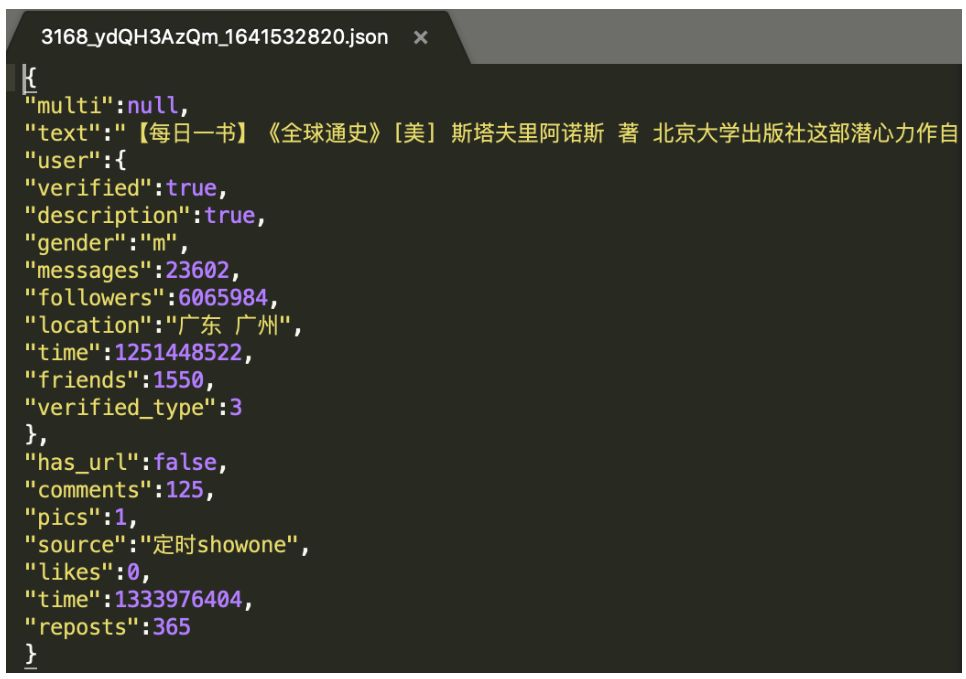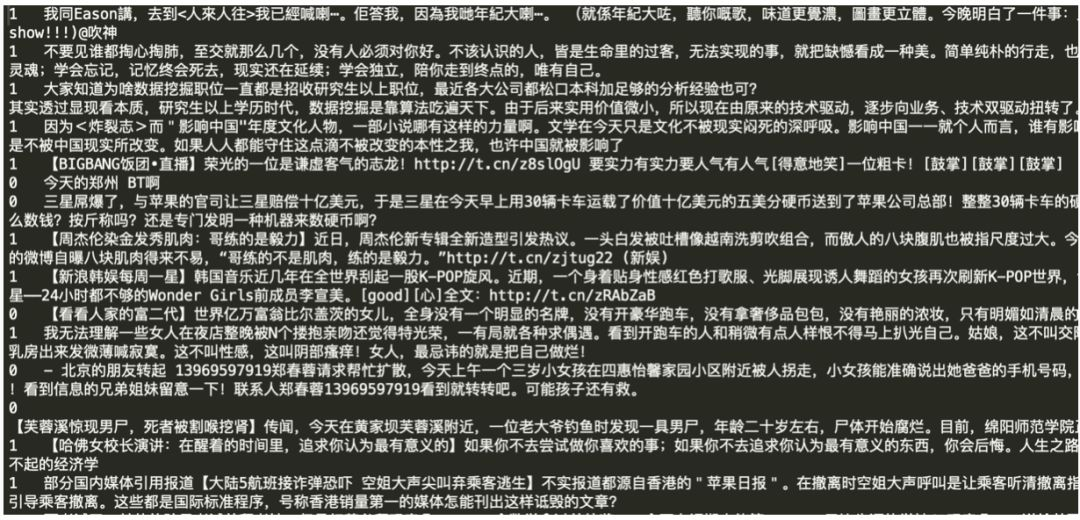#生成数据字典
def create_dict(data_path, dict_path):
dict_set = set()
# 读取全部数据
with open(data_path, 'r', encoding='utf-8') as f:
for line in lines:
content = line.split('\t')[-1].replace('\n', '')
for s in content:
# 把元组转换成字典，一个字对应一个数字
dict_list = []
i = 0
for s in dict_set:
dict_list.append([s, i])
i += 1
# 添加未知字符
dict_txt = dict(dict_list)
end_dict = {"<unk>": i}
dict_txt.update(end_dict)
# 把这些字典保存到本地中
with open(dict_path, 'w', encoding='utf-8') as f:
f.write(str(dict_txt))
print("数据字典生成完成！")
# 获取字典的长度
def get_dict_len(dict_path):
with open(dict_path, 'r', encoding='utf-8') as f:
return len(line.keys())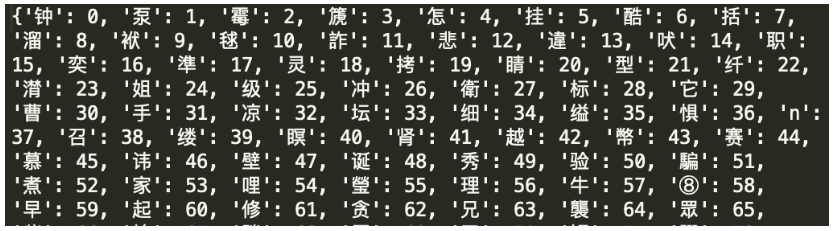# 创建序列化表示的数据,并按照一定比例划分训练数据与验证数据
def create_data_list(data_list_path):
#在生成数据之前，首先将eval_list.txt和train_list.txt清空
with open(os.path.join(data_list_path, 'eval_list.txt'), 'w', encoding='utf-8') as f_eval:
f_eval.seek(0)
f_eval.truncate()

with open(os.path.join(data_list_path, 'train_list.txt'), 'w', encoding='utf-8') as f_train:
f_train.seek(0)
f_train.truncate()

with open(os.path.join(data_list_path, 'dict.txt'), 'r', encoding='utf-8') as f_data:

with open(os.path.join(data_list_path, 'all_data.txt'), 'r', encoding='utf-8') as f_data:

i = 0
with open(os.path.join(data_list_path, 'eval_list.txt'), 'a', encoding='utf-8') as f_eval,open(os.path.join(data_list_path, 'train_list.txt'), 'a', encoding='utf-8') as f_train:
for line in lines:
words = line.split('\t')[-1].replace('\n', '')
label = line.split('\t')
labs = ""
if i % 8 == 0:
for s in words:
lab = str(dict_txt[s])
labs = labs + lab + ','
labs = labs[:-1]
labs = labs + '\t' + label + '\n'
f_eval.write(labs)
else:
for s in words:
lab = str(dict_txt[s])
labs = labs + lab + ','
labs = labs[:-1]
labs = labs + '\t' + label + '\n'
f_train.write(labs)
i += 1
print("数据列表生成完成！")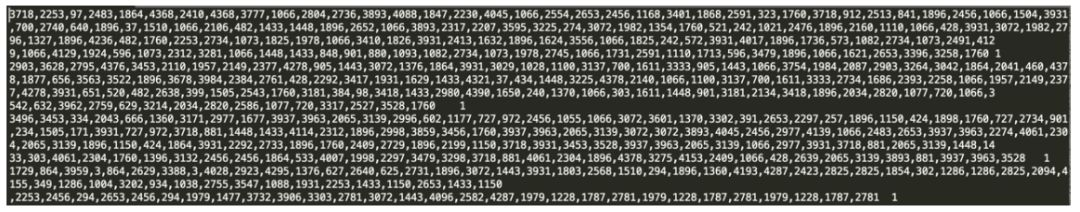def data_mapper(sample):
data, label = sample
data = [int(data) for data in data.split(',')]
return data, int(label)
#定义数据读取器
with open(data_path, 'r') as f:
for line in lines:
data, label = line.split('\t')
yield data, label



# 获取训练数据读取器和测试数据读取器
BATCH_SIZE = 128
train_list_path = data_list_path+'train_list.txt'
eval_list_path = data_list_path+'eval_list.txt'
batch_size= BATCH_SIZE)
batch_size= BATCH_SIZE)


（1）   模型定义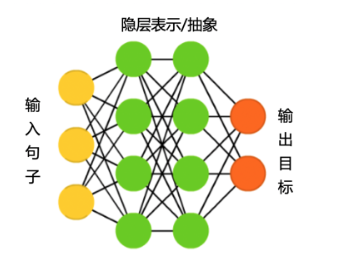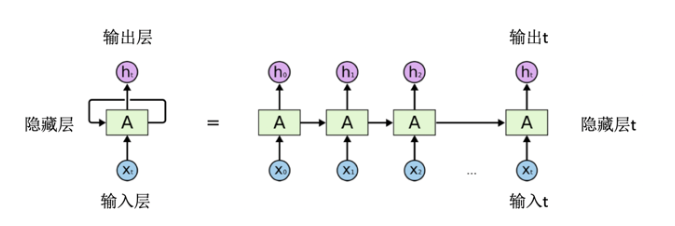# 定义长短期记忆网络
def lstm_net(ipt, input_dim):
# 以数据的IDs作为输入
emb = fluid.layers.embedding(input=ipt, size=[input_dim, 128], is_sparse=True)
# 第一个全连接层
fc1 = fluid.layers.fc(input=emb, size=128)
# 进行一个长短期记忆操作
lstm1, _ = fluid.layers.dynamic_lstm(input=fc1,
size=128)
# 第一个最大序列池操作
fc2 = fluid.layers.sequence_pool(input=fc1, pool_type='max')
# 第二个最大序列池操作
lstm2 = fluid.layers.sequence_pool(input=lstm1, pool_type='max')
# 以softmax作为全连接的输出层，大小为2
out = fluid.layers.fc(input=[fc2, lstm2], size=2, act='softmax')
return out



# 定义输入数据， lod_level不为0指定输入数据为序列数据
words = fluid.data(name='words', shape=[None,1], dtype='int64', lod_level=1)
label = fluid.data(name='label', shape=[None,1], dtype='int64')


# 获取数据字典长度
dict_dim = get_dict_len(dict_path)
# 获取分类器
model = lstm_net(words, dict_dim)


（2）损失函数

# 获取损失函数和准确率
cost = fluid.layers.cross_entropy(input=model, label=label)
avg_cost = fluid.layers.mean(cost)
acc = fluid.layers.accuracy(input=model, label=label)


test_program=fluid.default_main_program().clone(for_test=True)


（3）优化方法

# 定义优化方法
opt = optimizer.minimize(avg_cost)


fluid.default_startup_program：定义了创建模型参数，输入输出，以及模型中可学习参数的初始化等各种操作，由框架自动生成，使用时无需显示地创建；

fluid.default_main_program ：定义了神经网络模型，前向反向计算，以及优化算法对网络中可学习参数的更新，使用飞桨的核心就是构建起 default_main_program。

use_cuda = True
place = fluid.CUDAPlace(0) if use_cudaelse fluid.CPUPlace()#定义训练设备为CPU或GPU
exe = fluid.Executor(place)
exe.run(fluid.default_startup_program())     #执行参数初始化操作


feeder = fluid.DataFeeder(place=place,feed_list=[words, label])


EPOCH_NUM=50
model_save_dir = '/home/aistudio/work/infer_model/'
# 开始训练

for pass_id in range(EPOCH_NUM):
# 进行训练
train_cost, train_acc =  exe.run(program=fluid.default_main_program(),
feed=feeder.feed(data),
fetch_list=[avg_cost, acc])

if batch_id % 100 == 0:
print('Pass:%d, Batch:%d, Cost:%0.5f, Acc:%0.5f' % (pass_id, batch_id, train_cost, train_acc))
# 进行测试
eval_costs = []
eval_accs = []
eval_cost, eval_acc = exe.run(program=test_program,
feed=feeder.feed(data),
fetch_list=[avg_cost, acc])
eval_costs.append(eval_cost)
eval_accs.append(eval_acc)
# 计算平均预测损失在和准确率
eval_cost = (sum(eval_costs) / len(eval_costs))
eval_acc = (sum(eval_accs) / len(eval_accs))
print('Test:%d, Cost:%0.5f, ACC:%0.5f' % (pass_id, eval_cost, eval_acc))



model_save_dir = "/home/aistudio/work/model"
if not os.path.exists(model_save_dir):
os.makedirs(model_save_dir)
# 保存训练的模型，executor 把所有相关参数保存到 dirname 中
fluid.io.save_inference_model(model_save_dir,#保存模型的路径
[words.name],    #预测需要 feed 的数据
[model],    #保存预测结果的变量
exe)         #executor 保存预测模型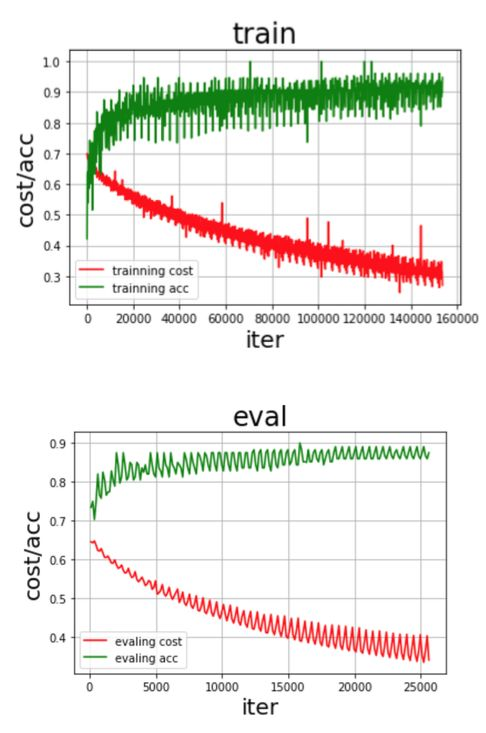# 用训练好的模型进行预测并输出预测结果
# 创建执行器
place = fluid.CPUPlace()
infer_exe = fluid.Executor(place)
infer_exe.run(fluid.default_startup_program())
save_path = '/home/aistudio/work/infer_model/'

# 从模型中获取预测程序、输入数据名称列表、分类器
[infer_program, feeded_var_names, target_var] =
# 获取数据
def get_data(sentence):
# 读取数据字典
with open('/home/aistudio/data/dict.txt', 'r', encoding='utf-8') as f_data:
dict_txt = dict(dict_txt)
# 把字符串数据转换成列表数据
keys = dict_txt.keys()
data = []
for s in sentence:
# 判断是否存在未知字符
if not s in keys:
s = '<unk>'
data.append(int(dict_txt[s]))
return data

data = []
# 获取预测数据
data1 = get_data('兴仁县今天抢小孩没抢走，把孩子母亲捅了一刀，看见这车的注意了，真事，车牌号辽HFM055！！！！！赶紧散播！都别带孩子出去瞎转悠了 尤其别让老人自己带孩子出去 太危险了 注意了！！！！辽HFM055北京现代朗动，在各学校门口抢小孩！！！110已经 证实！！全市通缉！！')
data2 = get_data('重庆真实新闻:2016年6月1日在重庆梁平县袁驿镇发生一起抢儿童事件，做案人三个中年男人，在三中学校到镇街上的一条小路上，把小孩直接弄晕(儿童是袁驿新幼儿园中班的一名学生)，正准备带走时被家长及时发现用棒子赶走了做案人，故此获救！请各位同胞们以此引起非常重视，希望大家有爱心的人传递下')
data3 = get_data('@尾熊C 要提前预习育儿知识的话，建议看一些小巫写的书，嘻嘻')
data.append(data1)
data.append(data2)
data.append(data3)

# 获取每句话的单词数量
base_shape = [[len(c) for c in data]]

# 生成预测数据
tensor_words = fluid.create_lod_ (data, base_shape, place)

# 执行预测
result = exe.run(program=infer_program,
feed={feeded_var_names: tensor_words},
fetch_list=target_var)

# 分类名称
names = [ '谣言', '非谣言']
# 获取结果概率最大的label
for i in range(len(data)):
lab = np.argsort(result)[i][-1]
print('预测结果标签为：%d， 分类为：%s， 概率为：%f' % (lab, names[lab], result[i][lab]))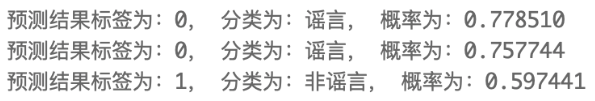下载安装命令

## CPU版本安装命令

## GPU版本安装命令
pip install -f https://paddlepaddle.org.cn/pip/oschina/gpu paddlepaddle-gpu### 作者的其它热门文章

0
0 收藏

0 评论
0 收藏
0